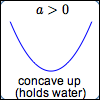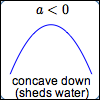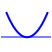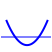﻿ Review of Quadratic Functions: Vertex Form, Max/Min, Intercepts, More

# REVIEW OF QUADRATIC FUNCTIONS: VERTEX FORM, MAX/MIN, INTERCEPTS, MORE

by Dr. Carol JVF Burns (website creator)
Follow along with the highlighted text while you listen!
Thanks for your support!
• PRACTICE (online exercises and printable worksheets)

DEFINITION quadratic function (standard form; vertex form)
Let $\,a\,$, $\,b\,$ and $\,c\,$ be real numbers, with $\,a \ne 0\,$.

A quadratic function is a function that can be written in the form $\ y = ax^2 + bx + c\$;
this is called the standard form of the quadratic function.

Every quadratic function can also be written in the form $\ y=a{(x-h)}^2 + k\$;
this is called the vertex form of the quadratic function.

For a thorough review of quadratic functions, including going from standard to vertex form,

## SUMMARY: PROPERTIES OF QUADRATIC FUNCTIONS

$y = ax^2 + bx + c$
(standard form)
$y = a(x-h)^2 + k$
(vertex form)
GRAPH Every quadratic function graphs as a parabola,
which has beautiful and important reflecting properties.If you want to know the focus and directrix of $\,y = a(x-h)^2 + k\,$,
For $\,a > 0\,$, $$\cssId{s22}{\underbrace{a(x-h)^2}_{\ge 0} + k \overset{\text{always!}}{\ge} k}$$ Thus, $\,k\,$ is a global minimum value.

For $\,a < 0\,$, $$\cssId{s25}{\underbrace{a(x-h)^2}_{\le 0} + k \overset{\text{always!}}{\le} k}$$ Thus, $\,k\,$ is a global maximum value.
VERTEX (turning point) (let $\,f(x) = ax^2 + bx + c\,$)

$\displaystyle\,\left(-\frac{b}{2a}\ ,\ f\bigl(-\frac{b}{2a}\bigr)\right)$

This is sometimes called the vertex formula, and is worth memorizing.
It can be derived by completing the square;
or, it's really easy with a bit of calculus!
$(h,k)$

Note how easy it is to get the vertex
from this form;

hence the name ‘vertex form’ is appropriate.
there is a horizontal tangent line
at the vertex
$\,y\,$-intercept when $\,x = 0\,$, $$\cssId{s39}{y = a\cdot 0^2 + b\cdot 0 + c = c}$$ when $\,x = 0\,$, $$\cssId{s41}{y = a(0 - h)^2 + k = ah^2 + k}$$ to find where a graph crosses the $\,y\,$-axis, set $\,x = 0\,$ and solve for $\,y$
$\,x\,$-intercept(s) use the Quadratic Formula,
as needed:

$ax^2 + bx + c = 0$

is equivalent to

$\displaystyle x = \frac{-b\pm\sqrt{b^2 - 4ac}}{2a}$$b^2 - 4ac = 0\,$ exactly one $\,x\,$-intercept $ax^2 + bx + c = 0$ has exactly one real number solution$b^2 - 4ac > 0\,$ two different $\,x\,$-intercepts $ax^2 + bx + c = 0$ has two different real number solutions$b^2 - 4ac < 0\,$ no $\,x\,$-intercepts $ax^2 + bx + c = 0$ has no real number solutions; it has two different complex number solutions
easiest:
isolate the squared expression;
correctly ‘undo’ the square

EXAMPLE: $$\begin{gather} \cssId{s66}{0 = 2(x-1)^2 -5}\cr\cr \cssId{s67}{5 = 2(x-1)^2}\cr\cr \cssId{s68}{\frac 52 = (x-1)^2}\cr\cr \cssId{s69}{x-1 = \pm\sqrt{\frac{5}{2}}} \cr\cr \cssId{s70}{x = 1\pm\sqrt{\frac{5}{2}}} \end{gather}$$ Need help with the last couple steps?

Study:

to find where a graph crosses the $\,x\,$-axis, set $\,y = 0\,$ and solve for $\,x$

Use the following Wolfram Alpha widgets to graph and compute properties of quadratic functions.
After pressing ‘Submit’ be sure to scroll down to see the graphs!

Master the ideas from this section
by practicing the exercise at the bottom of this page.

When you're done practicing, move on to:
max/min problems resulting in quadratic functions

On this exercise, you will not key in your answer.
However, you can check to see if your answer is correct.
PROBLEM TYPES:
 1 2 3 4 5 6 7 8
AVAILABLE MASTERED IN PROGRESS
 (MAX is 8; there are 8 different problem types.)